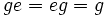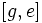# Trivial subgroup is normal

This article gives the statement, and possibly proof, of a subgroup property (i.e., normal subgroup) satisfying a subgroup metaproperty (i.e., trivially true subgroup property)
View all subgroup metaproperty satisfactions | View all subgroup metaproperty dissatisfactions |Get help on looking up metaproperty (dis)satisfactions for subgroup properties
Get more facts about normal subgroup |Get facts that use property satisfaction of normal subgroup | Get facts that use property satisfaction of normal subgroup|Get more facts about trivially true subgroup property

## Statement

Let$G$ be any group. The trivial subgroup of$G$, which is the one-element subgroup comprising the identity element, is a normal subgroup of$G$.

## Proof

### Conjugation definition of normality

Given: A group$G$ with identity element$e$. An element$g \in G$.

To prove:$geg^{-1} = e$.

Proof: We have$geg^{-1} = gg^{-1} = e$, completing the proof. Further information: manipulating equations in groups

### Kernel of homomorphism definition of normality

Given: A group$G$ with identity element$e$.

To prove: There exists a homomorphism$\varphi:G \to K$ for some group$K$ such that the kernel of$\varphi$ is precisely the subgroup$\{ e \}$.

Proof: Take$\varphi$ to be the identity map (i.e.,$\varphi(x) = x$ for all$x \in G$). This clearly satisfies the conditions for a homomorphism:$\varphi(gh) = gh = \varphi(g)\varphi(h)$,$\varphi(g^{-1}) = g^{-1} = \varphi(g)^{-1}$, and$\varphi(e) = e$.

The kernel of$\varphi$ is defined as the set of elements that map to$e$. But since this is the identity map, the set is precisely$\{ e \}$, completing the proof.

### Coset definition of normality

Given: A group$G$ with identity element$e$.

To prove: For any$g \in G$,$g \{ e \} = \{ e \} g$.

Proof: Both are clearly the same as the singleton set$\{ g \}$, because$ge = eg = g$.

### Union of conjugacy classes definition of normality

Given: A group$G$ with identity element$e$

To prove:$\{ e \}$ is a union of conjugacy classes.

Proof: In fact,$\{ e \}$ is a single conjugacy class, because for any$g \in G$,$geg^{-1} = e$.

### Commutator definition of normality

Given: A group$G$, with identity element$e$.

To prove: If$T = \{ e \}$, then$[G,T]$ is contained in$T$.

Proof:$[G,T]$ is the subgroup generated by elements of the form$[g,e]$, where$g \in G$. But$[g,e] = geg^{-1}e^{-1} = gg^{-1} = e$. Thus, all elements of the form$[g,e]$ equal$e$, so the subgroup generated is equal to$T$.